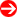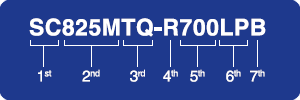#Product Naming Convention - ChassisCharacter Representation Options
1st Prefix
• SC = Super Chassis (Model Number)
• CSE = Super Chassis (Part Number Prefix)
(SC and CSE are interchangeable)
2nd Type/Family 2.5" HDD Chassis 3.5" HDD Chassis Mobile Rack
1st Digit = Height
• 0 = Twin / Extra short
• 1 = 1U
• 2 = 2U
• 3 = 3U / Mid-Tower
• 4 = 4U / Tower
• 5 = 5U
• 6 = 6U
1st Digit = Category
• 5xx = Compact size
• 7xx = Tower / Workstation
• 8xx / 9xx = Rackable chassis
• xx6 = Storage chassis
• xx9 = Resource Optimized
1st Digit (default= "M")
2nd Digit = Generation 2nd Digit = Height
• 0 = Twin / Extra short
• 1 = 1U
• 2 = 2U
• 3 = 3U / Mid-Tower
• 4 = 4U / Tower
• 5 = 5U
• 6 = 6U
2nd Digit = # of 5.25" Bays
3rd Digit = Category
• 1 = Cost Effective Series
• 2 = Standard Series
• 3 = High-end Series
• 6 = Storage Series
• 8 = MP motherboard Series
• 9 = Resource Optimized
3rd Digit = Generation 3rd Digit = # of 2.5" HDD Converted
4th Digit = Type (multiple types possible)
• (none) = Regular
• E = Lower Cost (Economic)
• F = Modified Fan (originally use blower)
• H = Specialized for Intel® Itanium 2
• L = Cost Effective
• M = Short-depth
• + = Specialized for AMD MBs (can be placed after backplane type)
4th Digit = Type (multiple types possible)
• (none) = Regular
• E = Lower Cost (Economic)
• F = Modified Fan (originally use blower)
• H = Specialized for Intel® Itanium 2
• L = Cost Effective
• M = Short-depth
• X = 10+ Slots
• + = Specialized for AMD MBs (can be placed after backplane type)
3rd Backplane Type
• (none) = No backplane design
• A = SAS2/SATA3 with iPass direct-attach connection
• AC(#) = SAS3/SATA3 & (#) of NVMe drives with Mini-SAS HD direct-attach connection
• E1/E16 = SAS(2)/SATA(3) with 1 expander
• E2/E26 = SAS(2)/SATA(3) with 2 expanders
• E1C = SAS3/SATA3 with 1 expander
• E2C = SAS3/SATA3 with 2 expanders
• H / HD / HQ = Hot-swap MB nodes design
• i = No backplane SKU
• S / S1 = SCSI (Single-Channel)
• S2 = SCSI (Dual-Channel)
• T = SAS / SATA
• TQ = SAS / SATA with SES2 support
• TS = Backplane not installed
• TG / G = GPU/Intel® Xeon Phi™ coprocessor optimized
4th Power Supply
• R = Redundant power supply
• (none) = Non-redundant power supply
5th Power Supply Wattage
• (example: 900 = 900 Watts)
6th Rear Window I/O
• (none) = Optimized (may not be standard)
• C = Standard I/O Rear Window
• LP = Low Profile Rear Window
• RC = Rear Window for Riser Cards, Type I
• RC2 = Rear Window for Riser Cards, Type II
• U = UIO Rear Window
7th Chassis Color
• (none) = Beige
• B = Black
• V = Silver